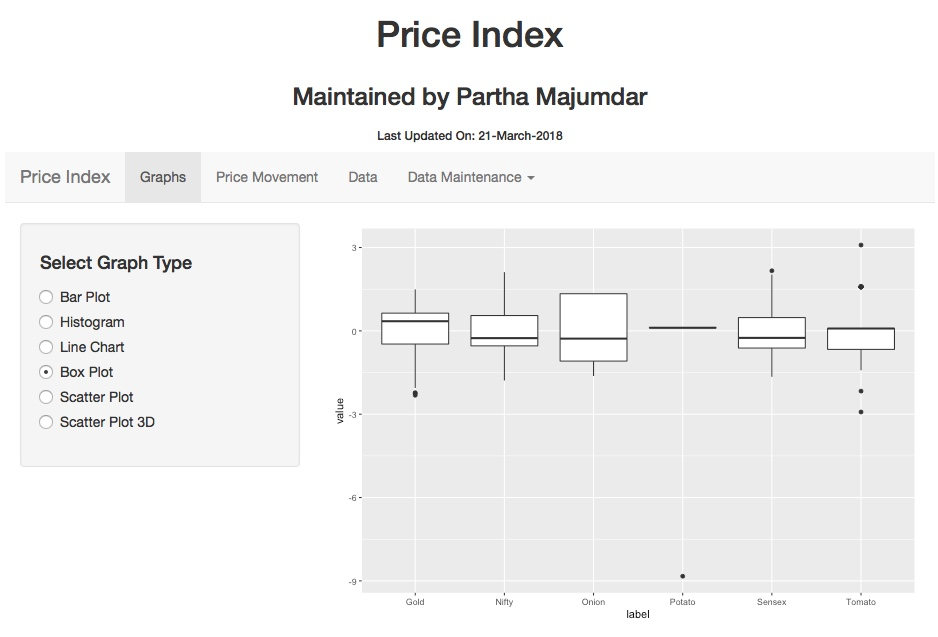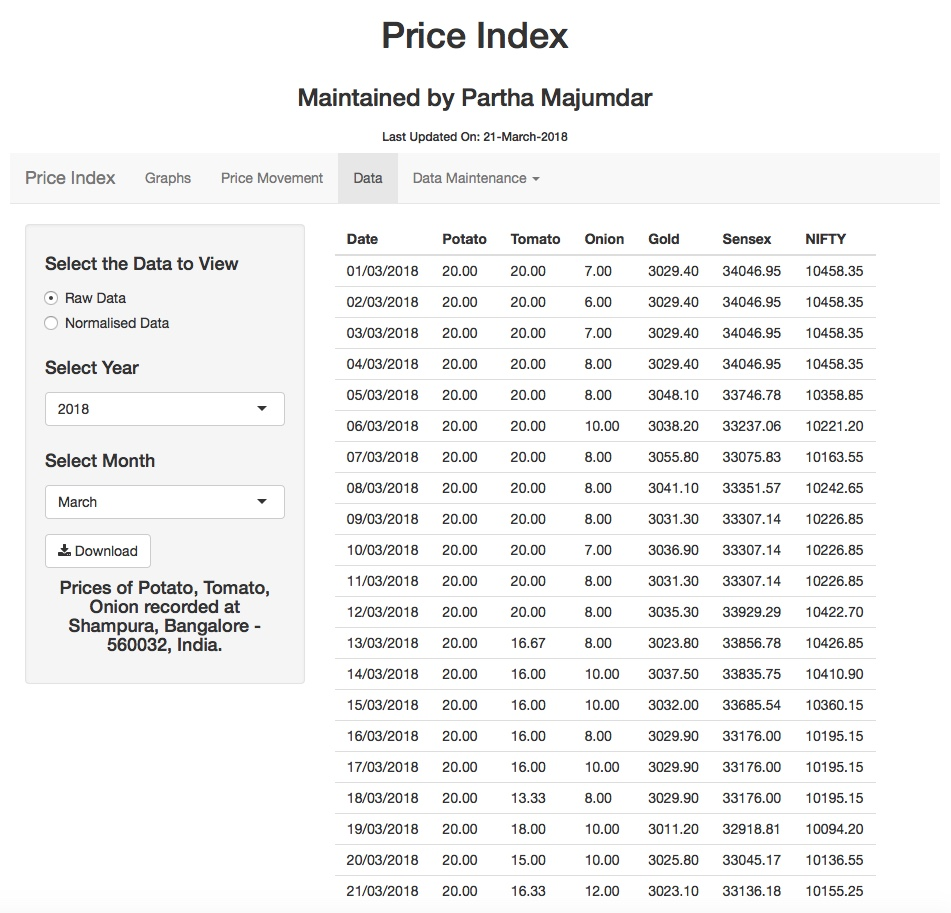Technical

# Programming with Shiny

Maintaining Data on Excel is becoming very difficult as the data is growing. Excel has become very slow as a result. Though my Mac has 16 GB of RAM, it takes between 2-3 minutes to save my Excel Workbook. So, I started making Applications and storing the data in Database. I have taken space in IBM Cloud for DB2 Database.

I spent the 2 days to make my Price Index Application. I programmed on Shiny for the first time and so had to frequently refer for syntax, etc. Shiny was my choice as it is easy to integrate R Language code in Shiny and present the output as a web page. I hosted my application on the R Studio server.

The application can be found at this URL – PriceIndex. It is also available from within this site under the Menu Option “Applications“.

The graphs do not make much sense now as the data set is small. However, as the data grows, I will add predictive analytics. Then, the site will recommend how prices of Stock Market and Gold will behave in future.

Also, I will next make this available as a Mobile App.

While writing this application, I came up some code snippets which can be useful for others trying to write applications in Shiny.

I used the following libraries to create this application. Note the syntax to installing the package if it is already not present in the environment.

```if("shiny" %in% rownames(installed.packages()) == FALSE) {install.packages("shiny")}
library(shiny)

if("ggplot2" %in% rownames(installed.packages()) == FALSE) {install.packages("ggplot2")}
library(ggplot2)

if("scatterplot3d" %in% rownames(installed.packages()) == FALSE) {install.packages("scatterplot3d")}
library(scatterplot3d)

if("lubridate" %in% rownames(installed.packages()) == FALSE) {install.packages("lubridate")}
library(lubridate)
```

I used RJDBC to connect to the DB2 database on IBM Cloud. To connect to the database, the following code was used. Note the use of dyn.load. It is absolutely required.

Also, find the path of db2jcc4.jar on your machine and provide the full path.

```dyn.load('/Library/Java/JavaVirtualMachines/jdk-9.0.4.jdk/Contents/Home/lib/server/libjvm.dylib')
library(rJava)
library(RJDBC)

#Enter the values for you database connection
dsn_driver = "com.ibm.db2.jcc.DB2Driver"
dsn_database = "BLUDB"            # e.g. "BLUDB"
dsn_hostname = "dashdb-entry-yp-lon02-01.services.eu-gb.bluemix.net"
dsn_port = "50000"
dsn_protocol = "TCPIP"
dsn_uid = "<em>user_id</em>"

jcc = JDBC("com.ibm.db2.jcc.DB2Driver", "<em>path</em>/db2jcc4.jar");
jdbc_path = paste("jdbc:db2://",  dsn_hostname, ":", dsn_port, "/", dsn_database, sep="");
conn = dbConnect(jcc, jdbc_path, user=dsn_uid, password=dsn_pwd)

query = "SELECT * FROM PRICEINDEX";
rs = dbSendQuery(conn, query);
df = fetch(rs, -1);
```

I use a validation to allow entry of prices as long as they are within 3 standard deviations from the mean. The maximum and minimum allowed values can be calculated as follows.

```number_of_sd <- 3
minGold <- max(1, round(mean(df\$Gold) - (number_of_sd * sd(df\$Gold))));
maxGold <- round(mean(df\$Gold) + (number_of_sd * sd(df\$Gold)));
```

The code to create the User Interface is as follows. A number of layouts and panels have been used. You can check the way to use them. Also, notice that controls have been created at runtime using uiOutput() function.

```ui <- fluidPage(

# Application title
fluidRow(
column(width = 12, align = "center", h1("Price Index"))
),
fluidRow(
column(width = 12, align = "center", h3("Maintained by Partha Majumdar"))
),
fluidRow(
column(width = 12, align = "center", h6(paste("Last Updated On:", "21-March-2018")))
),

navbarPage("Price Index",
tabPanel("Graphs",
# Sidebar
sidebarLayout(
sidebarPanel(
choices = list("Bar Plot" = 1,
"Histogram" = 2,
"Line Chart" = 3,
"Box Plot" = 4,
"Scatter Plot" = 5,
"Scatter Plot 3D" = 6),
selected = 4),
uiOutput("optionSelect"),
uiOutput("subOptionSelect"),
uiOutput("scatterSelect2"),
uiOutput("scatterSelect3")
),

# Show a plot
mainPanel(
plotOutput("dispPlot")
)
)
),
tabPanel("Price Movement",
# Sidebar
sidebarLayout(
sidebarPanel(
fluidRow(column(12,
span(h5("Prices of Potato, Tomato, Onion coming DOWN is GREEN, going UP is RED, no mevement is WHITE."),align='Center')),
span(h5("Price of Gold going UP is GREEN, coming DOWN is RED, no movement is WHITE."),align='Center'),
span(h5("Index of Sensex and NIFTY going UP is GREEN, coming DOWN is RED, no movement is WHITE."),align='Center')
),
selectInput("ddlYearHTML", h4("Select Year"), factor(df\$Year), selected = TRUE, multiple = FALSE),
selectInput("ddlMonthHTML", h4("Select Month"), factor(df\$MonthName)),
fluidRow(column(12,
span(h4("Prices of Potato, Tomato, Onion recorded at Shampura, Bangalore - 560032, India."),align='Center')
))
),

# Show a plot
mainPanel(
fluidRow(column(12,
htmlOutput("dispHTML")
))
)
)

),
tabPanel("Data",
# Sidebar
sidebarLayout(
sidebarPanel(
radioButtons("rbDataType", h4("Select the Data to View"),
choices = list("Raw Data" = 1,
"Normalised Data" = 2),
selected = 1),
selectInput("ddlYear", h4("Select Year"), factor(df\$Year), selected = TRUE, multiple = FALSE),
selectInput("ddlMonth", h4("Select Month"), factor(df\$MonthName)),
fluidRow(column(12,
span(h4("Prices of Potato, Tomato, Onion recorded at Shampura, Bangalore - 560032, India."),align='Center')
))
),

# Show a plot
mainPanel(
tableOutput("dispData")
)
)

),
fluidRow(
column(3,
h5("Enter the Date for which you want to record the Prices. By default, today's date id entered. Date cannot be more than Today's Date."),
h5("Enter the prices of the different commodities. By detault, the previous days prices are displayed."),
h5("Click on Save button to save the prices")
),
column(9,
fluidRow(column(6,
dateInput("diDate", "Enter Date of Observation", Sys.Date(), max = Sys.Date())
),
column(3,
h5(paste("Should be less than",Sys.Date(),sep = " "))
)
),
fluidRow(column(6,
numericInput("niPotato", "Enter Price of Potato per KG", subset(df, df\$Date == max(df\$Date))\$Potato, min = minPotato, max = maxPotato, step = 1)
),
column(3,
h5(paste("Range:",minPotato,"-",maxPotato))
)
),
fluidRow(column(6,
numericInput("niTomato", "Enter Price of Tomato per KG", subset(df, df\$Date == max(df\$Date))\$Tomato, min = minTomato, max = maxTomato, step = 1)
),
column(3,
h5(paste("Range:",minTomato,"-",maxTomato))
)
),
fluidRow(column(6,
numericInput("niOnion", "Enter Price of Onion per KG", subset(df, df\$Date == max(df\$Date))\$Onion, min = minOnion, max = maxOnion, step = 1)
),
column(3,
h5(paste("Range:",minOnion,"-",maxOnion))
)
),
fluidRow(column(6,
numericInput("niGold", "Enter Price of Gold per Gram", subset(df, df\$Date == max(df\$Date))\$Gold, min = minGold, max = maxGold, step = 1)
),
column(3,
h5(paste("Range:",minGold,"-",maxGold))
)
),
fluidRow(column(6,
numericInput("niSensex", "Enter SENSEX Index Value", subset(df, df\$Date == max(df\$Date))\$Sensex, min = minSensex, max = maxSensex, step = 1)
),
column(3,
h5(paste("Range:",minSensex,"-",maxSensex))
)
),
fluidRow(column(6,
numericInput("niNIFTY", "Enter NIFTY Index Value", subset(df, df\$Date == max(df\$Date))\$Nifty, min = minNIFTY, max = maxNIFTY, step = 1)
),
column(3,
h5(paste("Range:",minNIFTY,"-",maxNIFTY))
)
),
fluidRow(column(6,
),
column(3,
h5("Password is required to be able to save data")
)
),
actionButton(inputId = "sbSave", label = "Save")
)
),
fluidRow(
column(12,
h4(textOutput("DataEntry"))
)
)
),
tabPanel("Update",
fluidRow(
column(2,
),
column(10,
"Updatemain"
)
)),
plotOutput("dataUpdate")
)
)
)
```

First let us look at the data entry window. To save the data, the “Submit” button to be pressed. This event can be captured using the observeEvent() function. A snippet of the validation code is provided. After the validation, the ode for saving the record was written.

```  observeEvent(input\$sbSave, {
validRecord <- 0;
else {validRecord <- 0; msg <<- "Invalid Password"}
}

if(validRecord == 1) {
if(!is.null(input\$diDate) & length(input\$diDate) != 0) {
if(exists("oldRec")) {
if(nrow(oldRec) == 0) {
validRecord <- 1
}
else {
validRecord <- 0
}
}
else {
validRecord <- 0
msg <<- "Invalid Date provided"
}
}
else {
validRecord <- 0
msg < Sys.Date()) {
msg <<- "Date cannot be greater than Today"
validRecord <- 0
}
else {
validRecord <- 1
}
}

...

```

If you want to suppress the output of a part of the code, then put the code inside a sink block.

```          { sink("/dev/null");
newRecord <- data.frame(
Date = as.Date(input\$diDate, "%Y-%m-%d"),
Potato = input\$niPotato,
Tomato = input\$niTomato,
Onion = input\$niOnion,
Gold = input\$niGold,
Sensex = input\$niSensex,
Nifty = input\$niNIFTY
)
; sink(); }
```

By default, the variables in a code block are local. If you want the values of a variable to be available throughout the code, then use the `<<-` operator instead of `<-` operator.

```            dfDownload <<- rbind(dfDownload, newRecord)
```

To download the data into a Comma Separate Values file (CSV File), you can use the following code.

```output\$downloadData <- downloadHandler(

# This function returns a string which tells the client
# browser what name to use when saving the file.
filename = function() {
paste("PriceIndex", "csv", sep = ".")
},

# This function should write data to a file given to it by
# the argument 'file'.
content = function(file) {
# Write to a file specified by the 'file' argument
}
)
```

Based on event, if you would like your code to respond, you would need to program the reactive() function.

```  outputData <- reactive({
if(is.null(input\$ddlMonth)) {
df1 <- subset(df, df\$Year == input\$ddlYear)
}
else {
if(input\$ddlMonth == "") {
df1 <- subset(df, df\$Year == input\$ddlYear)
}
else {
df1 <- subset(df, df\$MonthName == input\$ddlMonth & df\$Year == input\$ddlYear)
}
}
...
}

output\$dispData <- renderTable({
outputData()
})
```

To build controls based on conditions, following code provides an example. Here, based on the type of Graph, the choices Radio Buttons are built dynamically.

```  choiceRadioButton <- reactive({
if(as.numeric(input\$rbGraphType) == 1 | as.numeric(input\$rbGraphType) == 2 | as.numeric(input\$rbGraphType) == 3 | as.numeric(input\$rbGraphType) == 5) {
choices = list("Potato" = 1,
"Tomato" = 2,
"Onion" = 3,
"Gold" = 4,
"Sensex" = 5,
"Nifty" = 6),
selected = 4)
}
}
)

...

output\$optionSelect <- renderUI({
})
```

To create the Bar Chart, the following code can be used. There are more features in the qplot function. I will update them later.

```  output\$dispPlot <- renderPlot({

if(as.numeric(input\$rbGraphType) == 1) {
#Bar Plot
if(is.null(input\$rbCommodity)) {
x <- data.matrix(df)
}
else {
x <- data.matrix(df[as.numeric(input\$rbCommodity) + 1])
}
qplot(x, geom = "bar", fill = I("yellow"), colour = I("red"))
}
```

To display the Histogram, the following code was used.

```      else if(as.numeric(input\$rbGraphType) == 2) {
#Histogram
if(is.null(input\$rbCommodity)) {
x <- data.matrix(df)
histTitle <- "Potato"
}
else {
x <- data.matrix(df[as.numeric(input\$rbCommodity) + 1])
histTitle <- switch(as.numeric(input\$rbCommodity), "Potato", "Tomato", "Onion", "Gold", "Sensex", "NIFTY")
}
histTitle <- paste("Histogram of", histTitle, "Prices between", as.character(min(df\$Date)), "and", as.character(max(df\$Date)), sep = " ")

# generate bins based on input\$bins from ui.R
if(is.null(input\$bins)) {
l <- 30
}
else {
l <- input\$bins
}
bins <- seq(min(x), max(x), length.out = l + 1)

# draw the histogram with the specified number of bins
hist(x, breaks = bins, col = 'darkgray', border = 'white', xlab = "Price Bins", ylab = "Frequency", main = histTitle)
}
```

To display the Line Chart, the following code was used.

```      else if(as.numeric(input\$rbGraphType) == 3) {
#Line Chart
if(is.null(input\$rbCommodity)) {
all_prices <- ggplot() +
geom_line(data = df,aes(x=c(1:nrow(df)), y = Potato), size=1, colour="brown") +
geom_line(data = df,aes(x=c(1:nrow(df)), y = Tomato), size =1, colour = "red") +
geom_line(data = df,aes(x=c(1:nrow(df)), y = Onion), size =1, colour = "purple") +
labs(x = "Date", y = "Price in Rs.")
print(all_prices)
}
...
```

To display the Box Plot, the following code was used.

```      else if(as.numeric(input\$rbGraphType) == 4) {
#Box Plot
dfBox <- data.frame(label = factor(rep(c("Potato","Tomato", "Onion", "Gold", "Sensex", "Nifty"), each=nrow(df))), value = c(df\$N_Potato, df\$N_Tomato, df\$N_Onion, df\$N_Gold, df\$N_Sensex, df\$N_Nifty))
ggplot(dfBox, aes(x=label, y=value)) + geom_boxplot()
}
```

To display the Scatter Plot, the following code was used.

```        ggplot(data = dfnew, aes(dfnew, dfnew, color = factor(MonthNum))) +
geom_point(aes(x = data.matrix(dfnew), y = data.matrix(dfnew))) +
scale_size_area() +
xlab(XLabel) +
ylab(YLabel) +
ggtitle(paste("Scatterplot of", XLabel, "vs.", YLabel, "and Month", sep = " "))
```

The user interfaces are shown below.